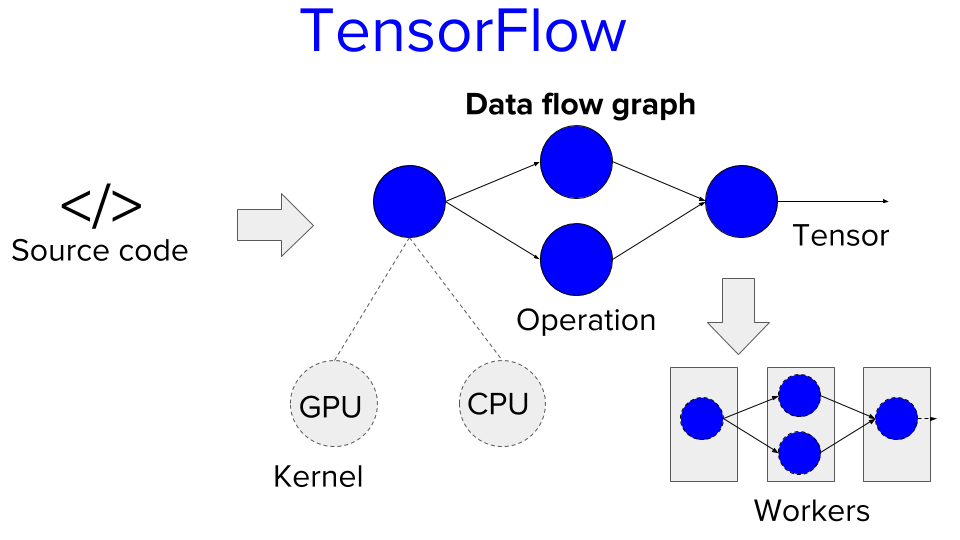[2-min CS Papers] A Short Introduction to the TensorFlow SystemMachine Learning (ML) is a sought-after skill in today’s automated world. Google is one of the key players in the Machine Learning space. With the growing scale and popularity of deep learning, the limitations of a single machine become more and more pronounced.

Google’s response to this challenge is the distributed TensorFlow system. TensorFlow is a Github project published in 2015 and described in the OSDI paper in 2016.

TensorFlow provides a high-level ML code library. Data scientists simply write code using the operations provided by the library. The TensorFlow system transforms this code into a data flow graph. Then it distributes the data flow graph to multiple machines and executes it in a distributed manner.

The data flow graph consists of operations and tensors. Each operation transforms ingoing to outgoing tensor data. Tensors are arrays or matrices of primitive data values. An example is the matrix multiplication operation. It receives two ingoing 2D matrices (tensors) and multiplies those to get the outgoing tensor.

TensorFlow provides hardware implementations for each abstract operation. The hardware implementation is denoted as a kernel. An operation may have different kernels for different hardware such as GPUs and CPUs.

One of the main languages to program against the TensorFlow API is Python. Want to learn Python? Visit our Finxter web app.